# Welcome to Physics 2135 PHYSICS 2135 Engineering Physics

• Slides: 51Welcome… …to Physics 2135.PHYSICS 2135 Engineering Physics II Dr. S. Thomas Vojta Instructor in charge Office: 204 Physics, Phone: 341 ‑ 4793 [email protected] edu www. mst. edu/~vojtat Office hours: Mon+ Wed 11 am-12 pm and by appointment Course website: Textbook: www. mst. edu/~vojtat/class_2135 University Physics with Modern Physics, Vol. 2 Young and Freedman, 14 th Edition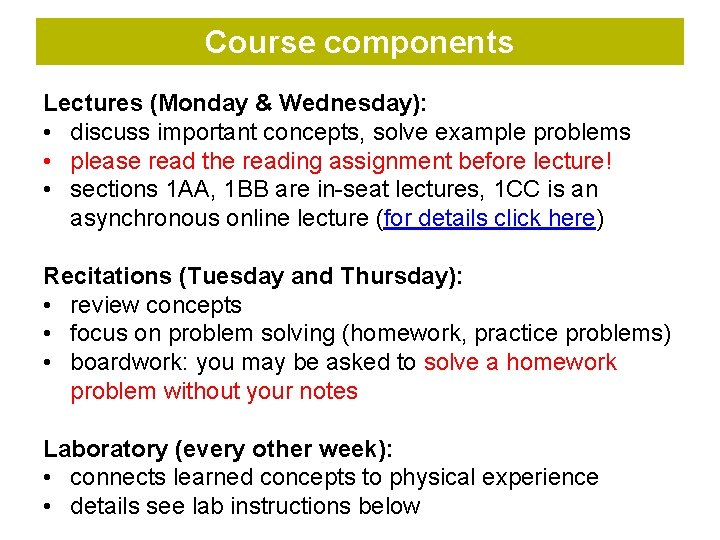Course components Lectures (Monday & Wednesday): • discuss important concepts, solve example problems • please read the reading assignment before lecture! • sections 1 AA, 1 BB are in-seat lectures, 1 CC is an asynchronous online lecture (for details click here) Recitations (Tuesday and Thursday): • review concepts • focus on problem solving (homework, practice problems) • boardwork: you may be asked to solve a homework problem without your notes Laboratory (every other week): • connects learned concepts to physical experience • details see lab instructions below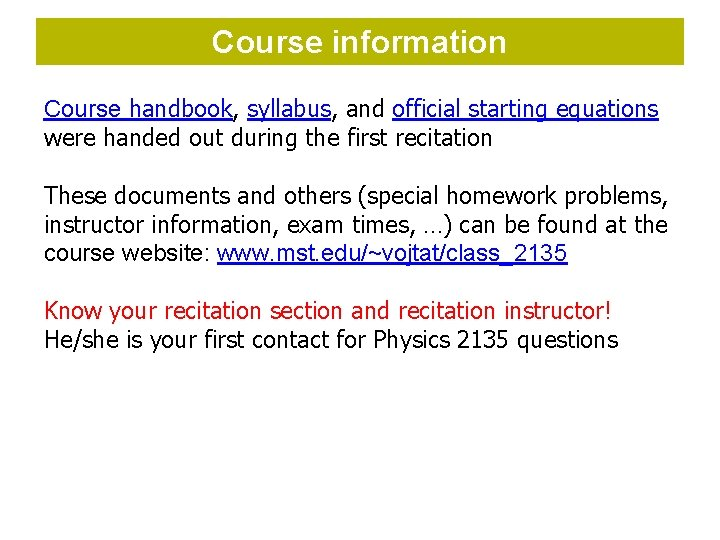Course information Course handbook, syllabus, and official starting equations were handed out during the first recitation These documents and others (special homework problems, instructor information, exam times, …) can be found at the course website: www. mst. edu/~vojtat/class_2135 Know your recitation section and recitation instructor! He/she is your first contact for Physics 2135 questions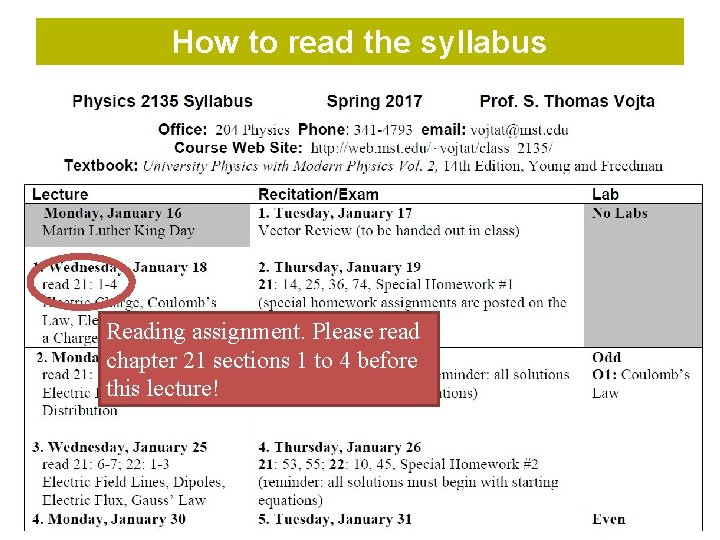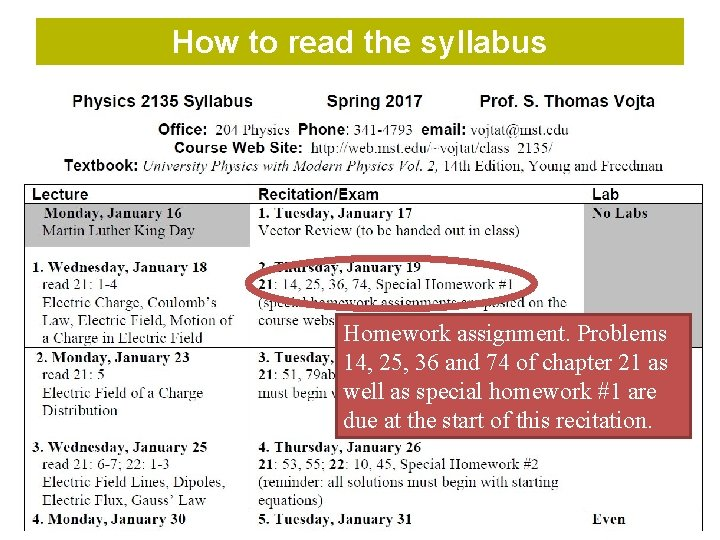How to read the syllabus Homework assignment. Problems 14, 25, 36 and 74 of chapter 21 as well as special homework #1 are due at the start of this recitation.How to read the syllabus Lab, odd and even refers to your lab section numberLaboratory information Laboratory website: http: //physics. mst. edu/currentcourses/labs/index. html Professor in charge of lab: Dr. Dan Waddill, [email protected] edu, 341 -4797, 103 Physics You must purchase a lab manual at the Physics Office, room 102, for \$25 (cash). You will not receive lab credit without a lab manual. Your lab section is numbered 3 L"some number". If "some number" is even, you are in EVEN labs. If "some number" is odd, you are in ODD labs. There are no labs this week!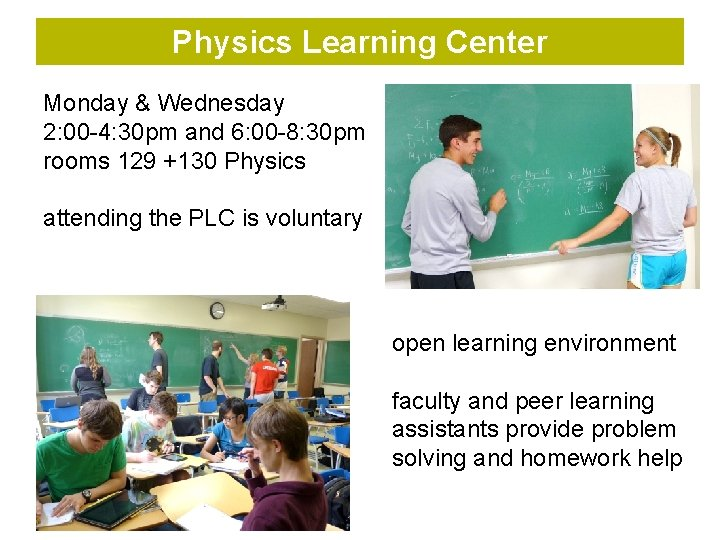Physics Learning Center Monday & Wednesday 2: 00 -4: 30 pm and 6: 00 -8: 30 pm rooms 129 +130 Physics attending the PLC is voluntary open learning environment faculty and peer learning assistants provide problem solving and homework help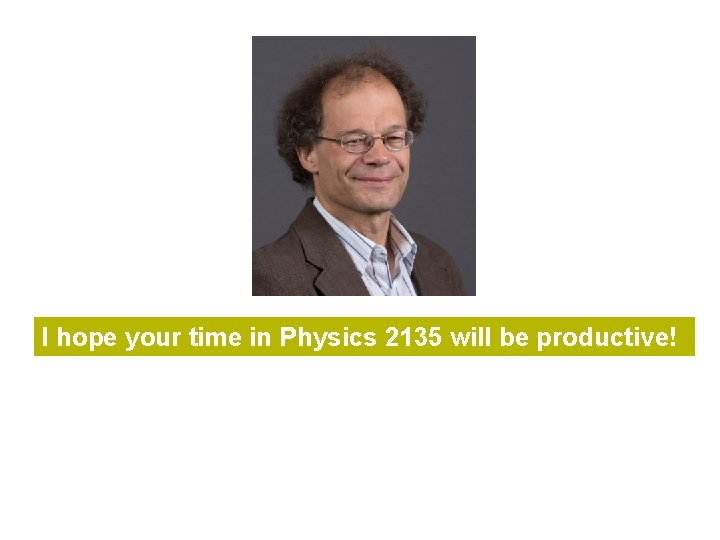I hope your time in Physics 2135 will be productive!Lecture 1 agenda: Electric Charge. Review of some things you hopefully learned in high school. Coulomb’s Law (electrical force between charged particles). You must be able to calculate the electrical forces between one or more charged particles. The electric field. You must be able to calculate the force on a charged particle in an electric field. Electric field due to point charges. You must be able to calculate electric field of one or more point charges. Motion of a charged particle in a uniform electric field. You must be able to solve for the trajectory of a charged particle in a uniform electric field.Electric Charge What is charge? • basic property of matter (just like, say, mass) • humans cannot directly sense charge but some animals can • we can observe charge indirectly via its effects on matter • customary symbol: q or Q, unit: [q] = C (Coulomb) Two kinds of charge: + - • like charges repel • unlike charges attract Law of conservation of charge: • net amount of charge does not change in any process (Not on your starting equation sheet, but a fact that you can use any time. )Charges are quantized (come in units of e= 1. 6 x 10 -19 C). Elementary particles that make up atoms: • Protons + charge +e = +1. 6 x 10 -19 C • Neutrons uncharged • Electrons charge –e = – 1. 6 x 10 -19 C - Helium atom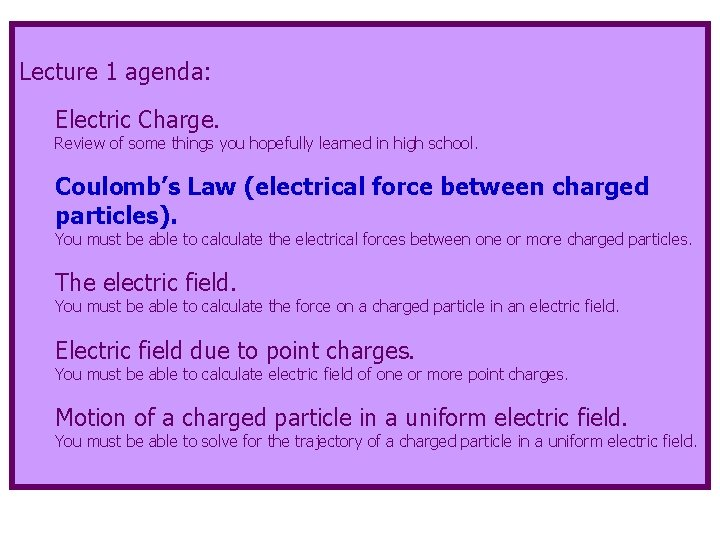Lecture 1 agenda: Electric Charge. Review of some things you hopefully learned in high school. Coulomb’s Law (electrical force between charged particles). You must be able to calculate the electrical forces between one or more charged particles. The electric field. You must be able to calculate the force on a charged particle in an electric field. Electric field due to point charges. You must be able to calculate electric field of one or more point charges. Motion of a charged particle in a uniform electric field. You must be able to solve for the trajectory of a charged particle in a uniform electric field.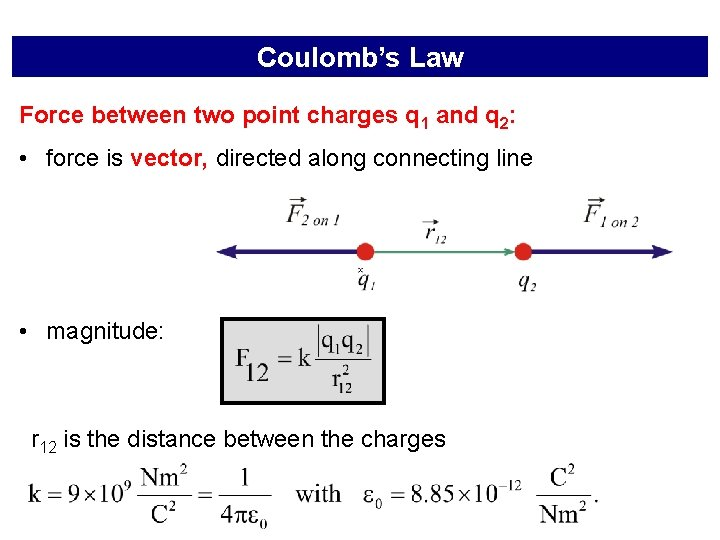Coulomb’s Law Force between two point charges q 1 and q 2: • force is vector, directed along connecting line • magnitude: r 12 is the distance between the chargesa note on starting equations is an official starting equation • in homework and exam solutions, official starting equations can be used without deriving them • all other steps of a solution need to be derived • you may formulate the official starting equation in different variables, • for example the equation is “legal” *“Starting” does not mean that a starting equation has to be the first thing that appears on your paper. It might be several lines before you use a starting equation.Coulomb’s Law is strictly valid for point charges only. It is a good approximation for small uniformly charged objects. r 12 - + If more than two charges are involved, the net force is the vector sum of all forces (superposition). For objects with complex shapes, you must add up all the forces acting on each separate charge (calculus!!). + + + - - -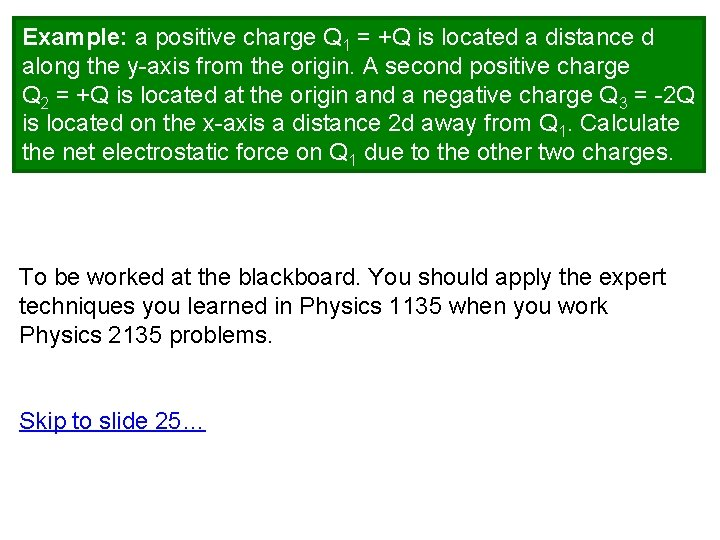Example: a positive charge Q 1 = +Q is located a distance d along the y-axis from the origin. A second positive charge Q 2 = +Q is located at the origin and a negative charge Q 3 = -2 Q is located on the x-axis a distance 2 d away from Q 1. Calculate the net electrostatic force on Q 1 due to the other two charges. To be worked at the blackboard. You should apply the expert techniques you learned in Physics 1135 when you work Physics 2135 problems. Skip to slide 25…Example: a positive charge Q 1 = +Q is located a distance d along the y-axis from the origin. A second positive charge Q 2 = +Q is located at the origin and a negative charge Q 3 = -2 Q is located on the x-axis a distance 2 d away from Q 1. Calculate the net electrostatic force on Q 1 due to the other two charges. y Q 1=+Q d Q 2=+Q 2 d Q 3=-2 Q x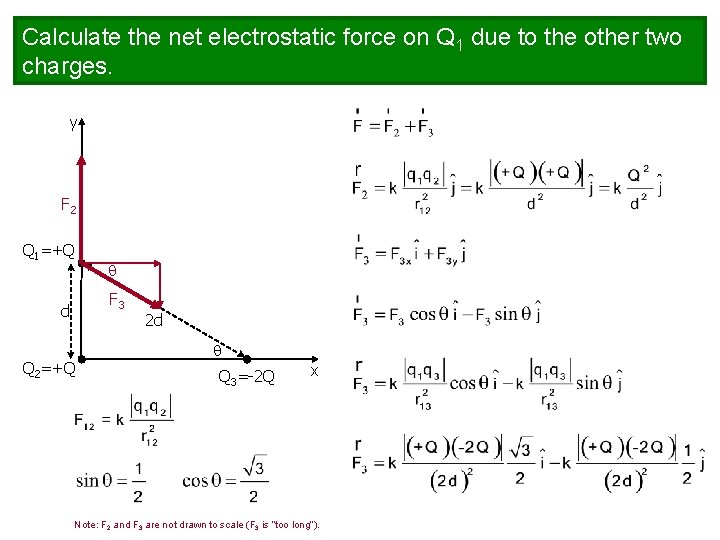Calculate the net electrostatic force on Q 1 due to the other two charges. y F 2 Q 1=+Q F 3 d Q 2=+Q 2 d Q 3=-2 Q x Note: F 2 and F 3 are not drawn to scale (F 3 is “too long”).Calculate the net electrostatic force on Q 1 due to the other two charges. y F 2 Q 1=+Q F 3 d Q 2=+Q 2 d Q 3=-2 Q x Note: F 2 and F 3 are not drawn to scale (F 3 is “too long”).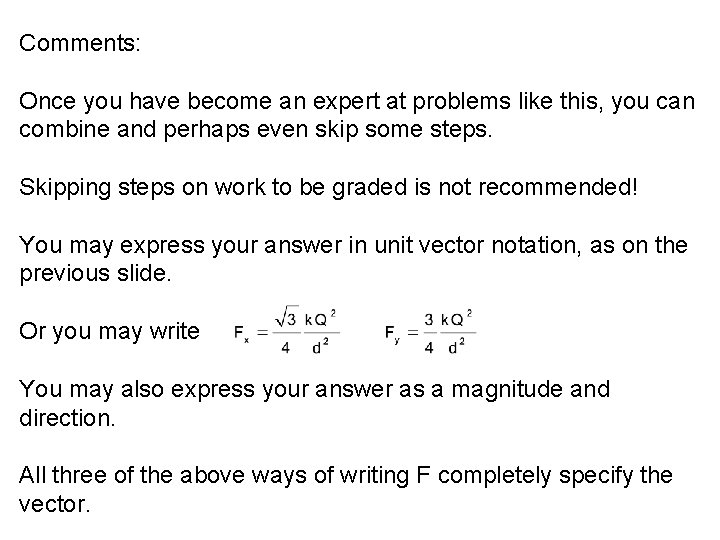Comments: Once you have become an expert at problems like this, you can combine and perhaps even skip some steps. Skipping steps on work to be graded is not recommended! You may express your answer in unit vector notation, as on the previous slide. Or you may write You may also express your answer as a magnitude and direction. All three of the above ways of writing F completely specify the vector.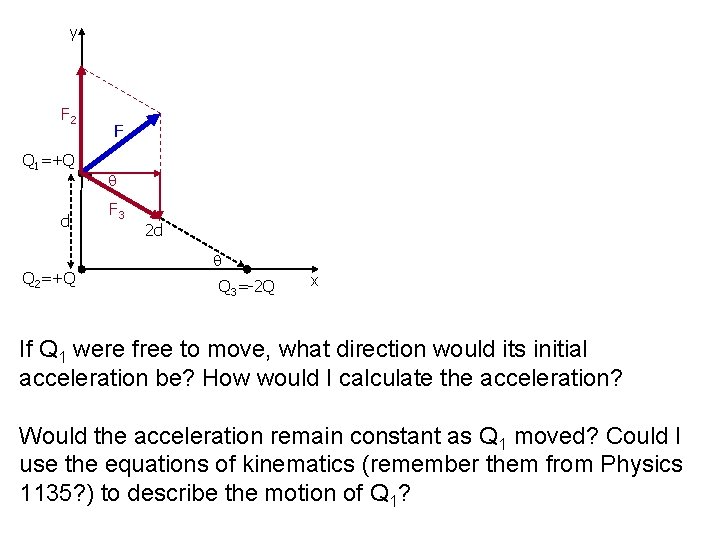y F 2 Q 1=+Q d Q 2=+Q F F 3 2 d Q 3=-2 Q x If Q 1 were free to move, what direction would its initial acceleration be? How would I calculate the acceleration? Would the acceleration remain constant as Q 1 moved? Could I use the equations of kinematics (remember them from Physics 1135? ) to describe the motion of Q 1?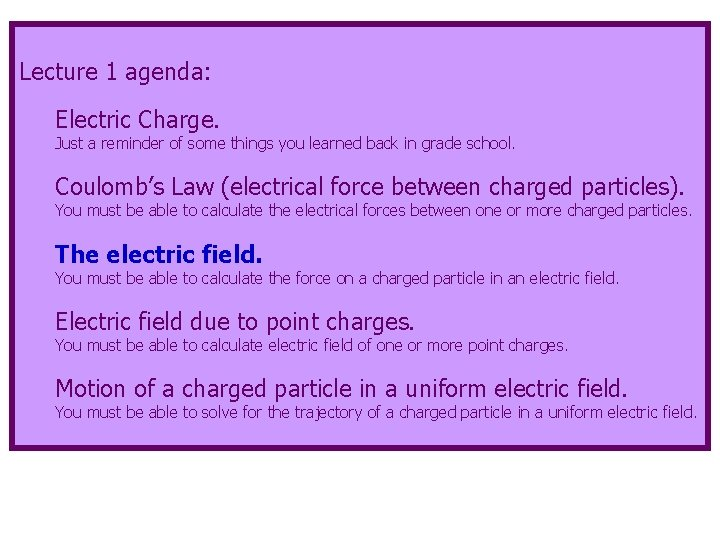Lecture 1 agenda: Electric Charge. Just a reminder of some things you learned back in grade school. Coulomb’s Law (electrical force between charged particles). You must be able to calculate the electrical forces between one or more charged particles. The electric field. You must be able to calculate the force on a charged particle in an electric field. Electric field due to point charges. You must be able to calculate electric field of one or more point charges. Motion of a charged particle in a uniform electric field. You must be able to solve for the trajectory of a charged particle in a uniform electric field.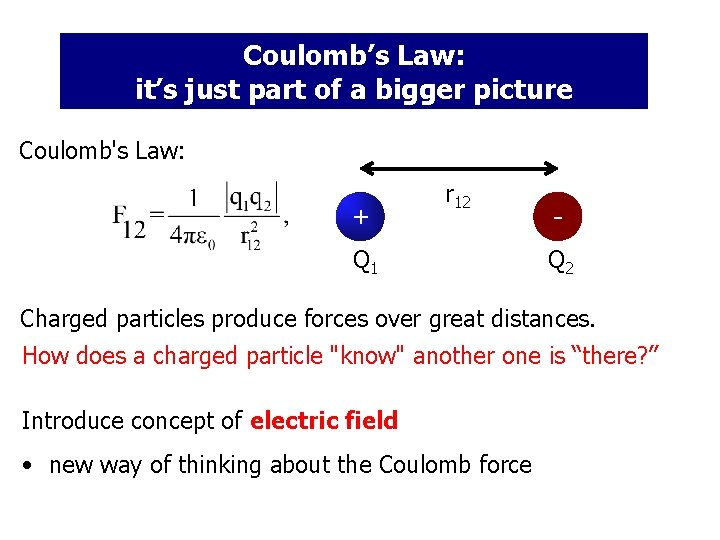Coulomb’s Law: it’s just part of a bigger picture Coulomb's Law: + r 12 Q 1 Q 2 Charged particles produce forces over great distances. How does a charged particle "know" another one is “there? ” Introduce concept of electric field • new way of thinking about the Coulomb force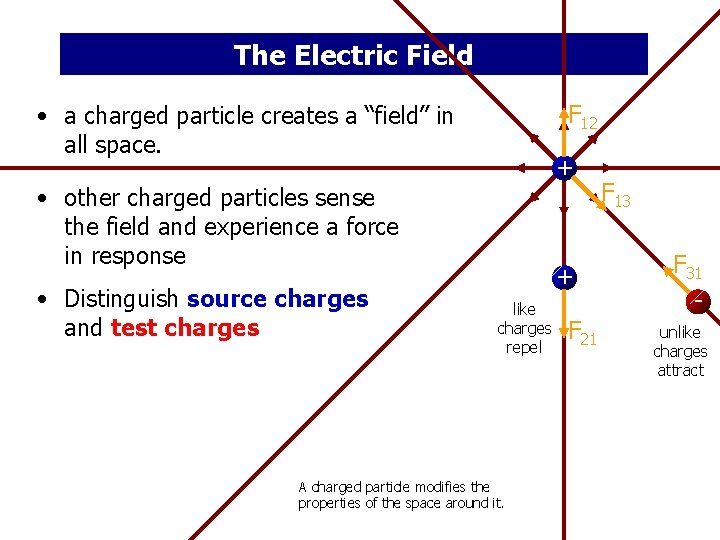The Electric Field F 12 • a charged particle creates a “field” in all space. + • other charged particles sense the field and experience a force in response • Distinguish source charges and test charges + like charges repel A charged particle modifies the properties of the space around it. F 21 F 13 F 31 unlike charges attractDefinition of electric field: • one or more source charges • define the electric field charge q 0: via force they exert on a test The subscript “ 0” reminds you the force is on the “test charge. ” I won’t require the subscripts when you use this equation for boardwork or on exams. This is your second starting equation. It is a vector equation that tells you magnitude and direction of the force!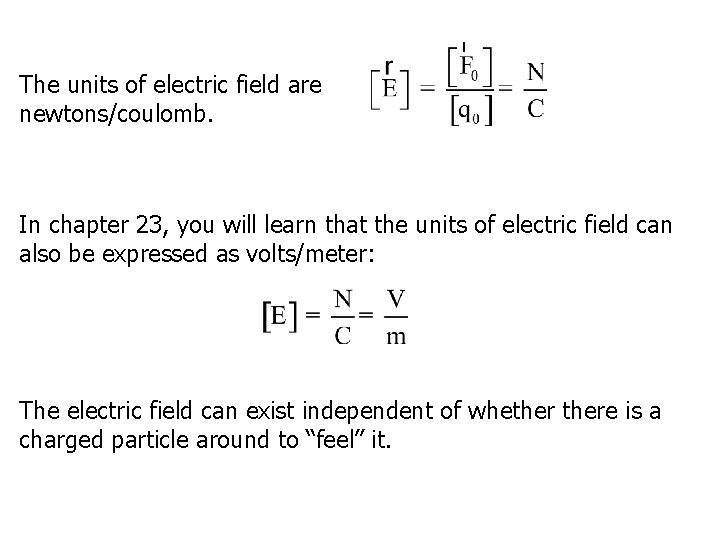The units of electric field are newtons/coulomb. In chapter 23, you will learn that the units of electric field can also be expressed as volts/meter: The electric field can exist independent of whethere is a charged particle around to “feel” it.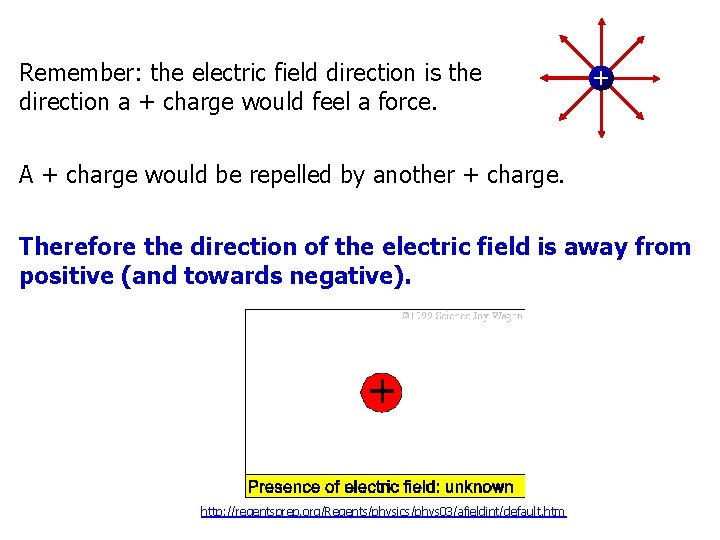Remember: the electric field direction is the direction a + charge would feel a force. + A + charge would be repelled by another + charge. Therefore the direction of the electric field is away from positive (and towards negative). http: //regentsprep. org/Regents/physics/phys 03/afieldint/default. htmGravitational Fields The idea of a field is not new to you. You experienced fields (gravitational) in Physics 1135. Units of g are actually N/kg! is the local gravitational field. On earth, it is about 9. 8 N/kg, directed towards the center of the earth. A particle with mass modifies the properties of the space around it.Lecture 1 agenda: Electric Charge. Review of some things you hopefully learned in high school. Coulomb’s Law (electrical force between charged particles). You must be able to calculate the electrical forces between one or more charged particles. The electric field. You must be able to calculate the force on a charged particle in an electric field. Electric field due to point charges. You must be able to calculate electric field of one or more point charges. Motion of a charged particle in a uniform electric field. You must be able to solve for the trajectory of a charged particle in a uniform electric field.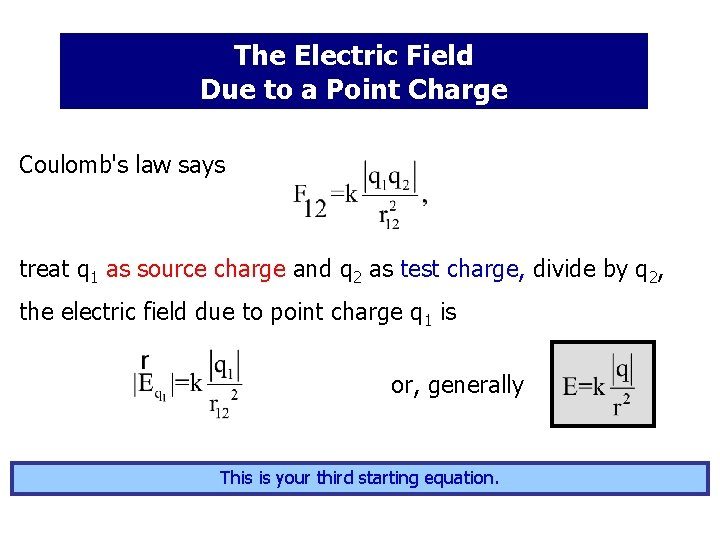The Electric Field Due to a Point Charge Coulomb's law says treat q 1 as source charge and q 2 as test charge, divide by q 2, the electric field due to point charge q 1 is or, generally This is your third starting equation.If we define point… as a unit vector from the source point to the field source point + field point …then the vector equation for the electric field of a point charge becomes: You may start with either equation for the electric field (this one or the on the previous slide). But only use this one if you REALLY know what you are doing!Example: calculate the magnitude of the electric field at the electron’s distance away from the proton in a hydrogen atom (5. 3 x 10 -11 m). +e + -e - EP D For comparison, air begins to break down and conduct electricity at about 30 k. V/cm, or 3 x 106 V/m.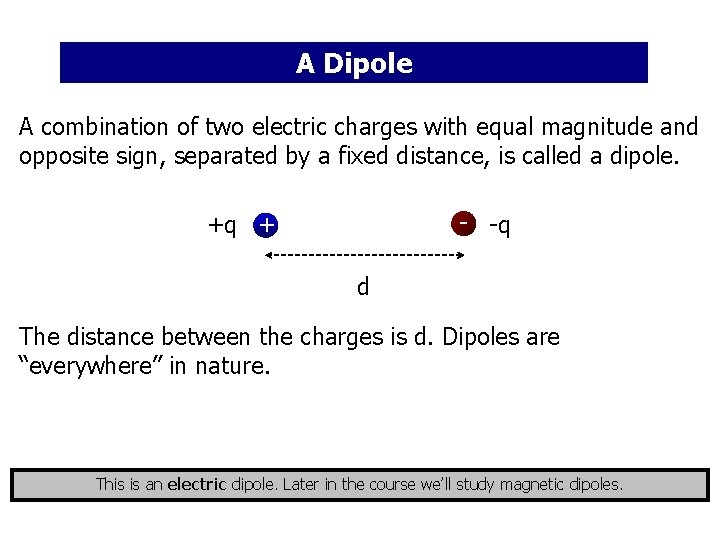A Dipole A combination of two electric charges with equal magnitude and opposite sign, separated by a fixed distance, is called a dipole. - -q +q + d The distance between the charges is d. Dipoles are “everywhere” in nature. This is an electric dipole. Later in the course we’ll study magnetic dipoles.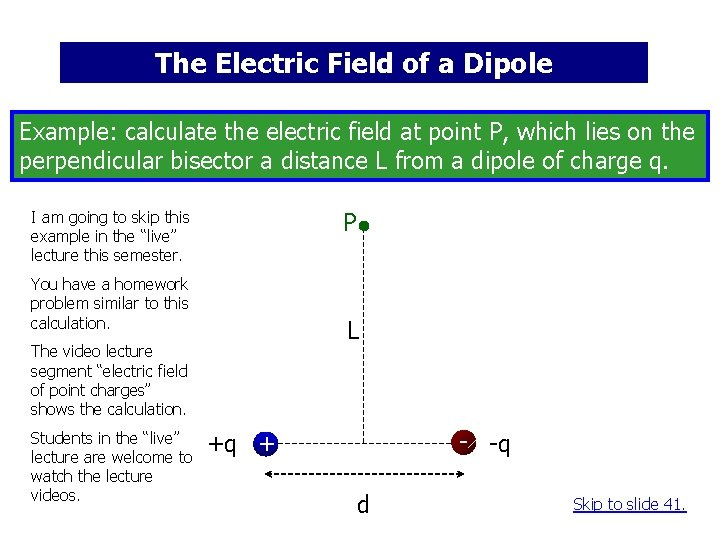The Electric Field of a Dipole Example: calculate the electric field at point P, which lies on the perpendicular bisector a distance L from a dipole of charge q. I am going to skip this example in the “live” lecture this semester. P You have a homework problem similar to this calculation. L The video lecture segment “electric field of point charges” shows the calculation. Students in the “live” lecture are welcome to watch the lecture videos. - -q +q + d Skip to slide 41.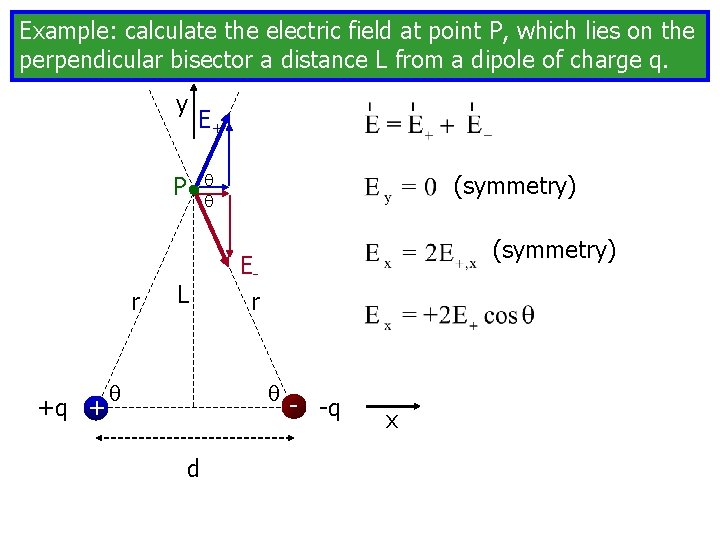Example: calculate the electric field at point P, which lies on the perpendicular bisector a distance L from a dipole of charge q. y E+ P r +q + L (symmetry) Er d - -q x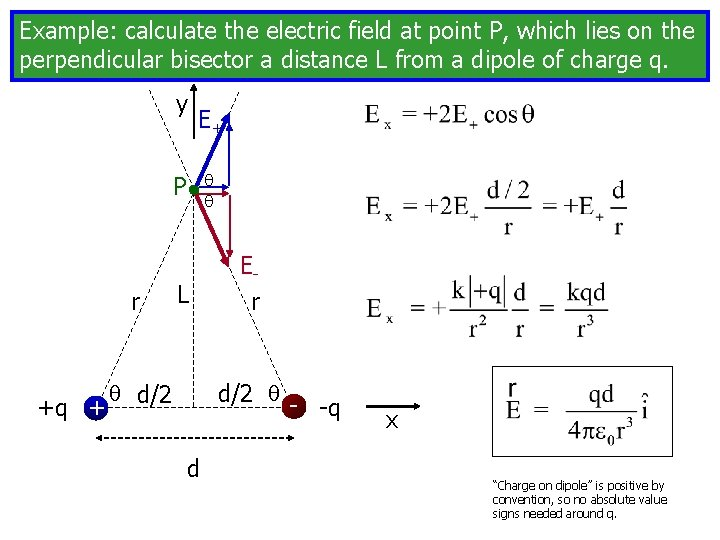Example: calculate the electric field at point P, which lies on the perpendicular bisector a distance L from a dipole of charge q. y E+ P r +q + L d/2 Er d/2 -q d x “Charge on dipole” is positive by convention, so no absolute value signs needed around q.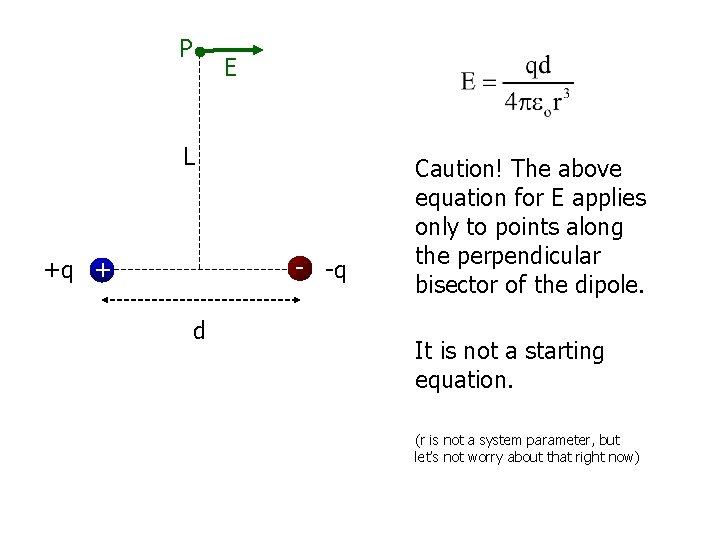P E L - -q +q + d Caution! The above equation for E applies only to points along the perpendicular bisector of the dipole. It is not a starting equation. (r is not a system parameter, but let’s not worry about that right now)Lecture 1 agenda: Electric Charge. Review of some things you hopefully learned in high school. Coulomb’s Law (electrical force between charged particles). You must be able to calculate the electrical forces between one or more charged particles. The electric field. You must be able to calculate the force on a charged particle in an electric field. Electric field due to point charges. You must be able to calculate electric field of one or more point charges. Motion of a charged particle in a uniform electric field. You must be able to solve for the trajectory of a charged particle in a uniform electric field.Motion of a Charged Particle in a Uniform Electric Field charged particle in electric field experiences force, and if it is free to move, an acceleration. if the only force is due to the electric field - - - - F E + + + + E is constant a constant, use the equations of kinematics* (Physics 1135? ). *If you get called to the board, you can use the Physics 1135 starting equations. They are posted.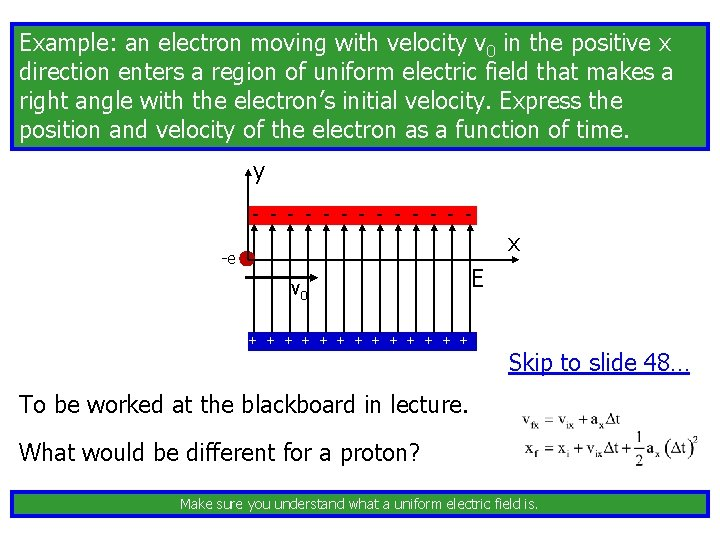Example: an electron moving with velocity v 0 in the positive x direction enters a region of uniform electric field that makes a right angle with the electron’s initial velocity. Express the position and velocity of the electron as a function of time. y - - - - x -e v 0 + + + + E Skip to slide 48… To be worked at the blackboard in lecture. What would be different for a proton? Make sure you understand what a uniform electric field is.Express the position and velocity of the electron as a function of time. y -e v 0 FE a E x Let’s work the rest of the problem one component at a time.Express the position and velocity of the electron as a function of time. y 0 -e v 0 FE a E x 0 Position: 0 0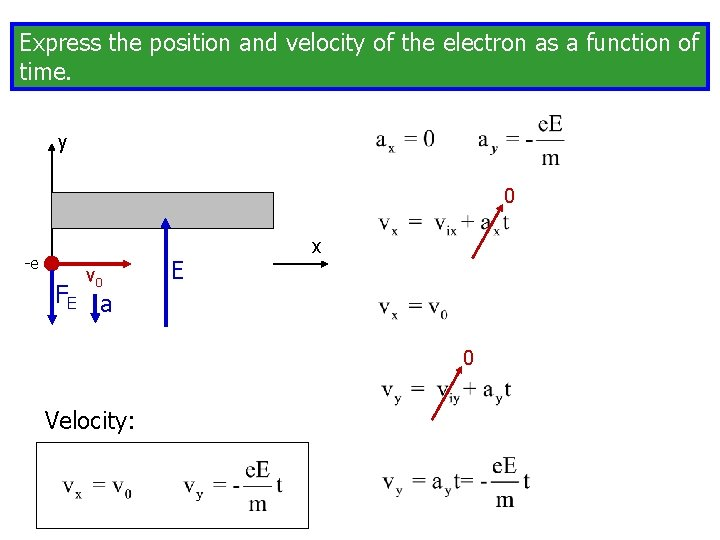Express the position and velocity of the electron as a function of time. y 0 -e v 0 FE a E x 0 Velocity: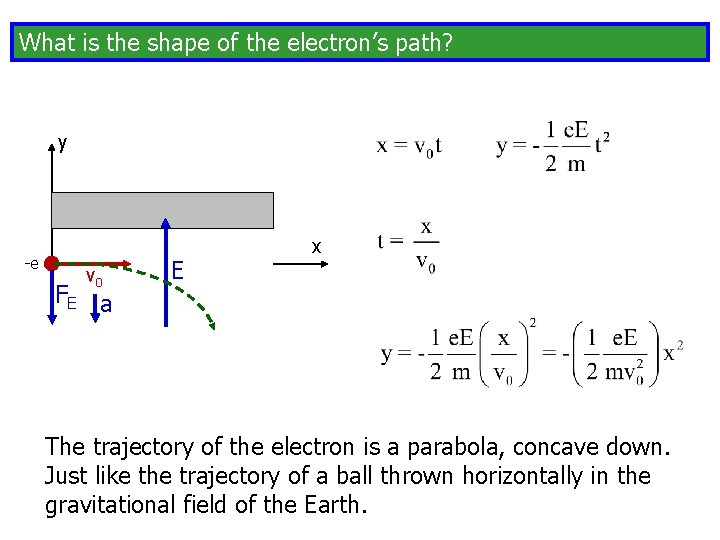What is the shape of the electron’s path? y -e v 0 FE a E x The trajectory of the electron is a parabola, concave down. Just like the trajectory of a ball thrown horizontally in the gravitational field of the Earth.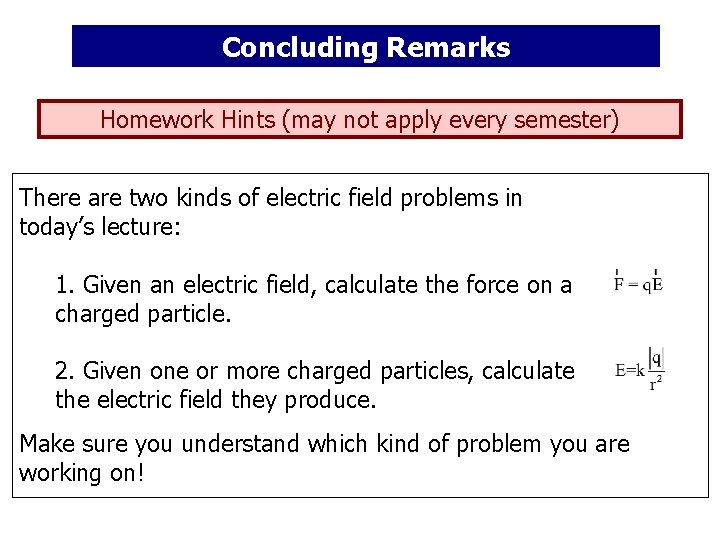Concluding Remarks Homework Hints (may not apply every semester) There are two kinds of electric field problems in today’s lecture: 1. Given an electric field, calculate the force on a charged particle. 2. Given one or more charged particles, calculate the electric field they produce. Make sure you understand which kind of problem you are working on!Homework Hints (may not apply every semester) Symmetry is your friend. Use it when appropriate. Don’t use it when not appropriate. The above equation is on the Physics 1135 Starting Equation Sheet, which is posted in the recitation classrooms. You are free to use Physics 1135 starting equations at any time.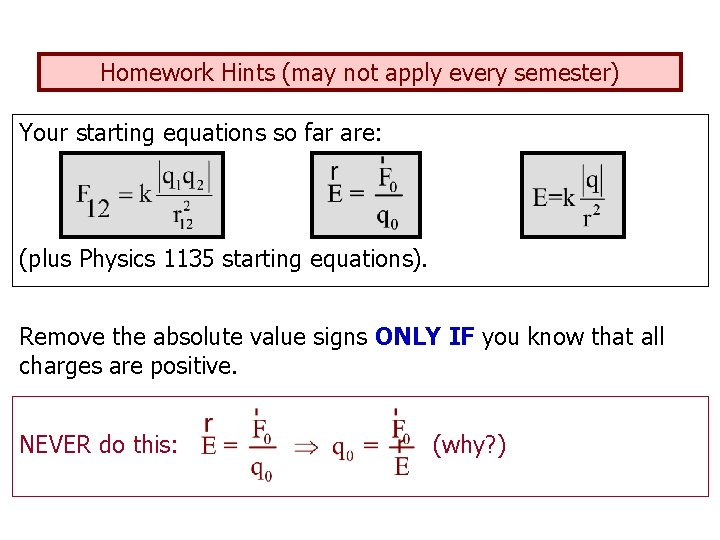Homework Hints (may not apply every semester) Your starting equations so far are: (plus Physics 1135 starting equations). Remove the absolute value signs ONLY IF you know that all charges are positive. NEVER do this: (why? )Learning Center Today 2: 00 -4: 30, 6: 00 -8: 30 Rooms 129/130 Physics